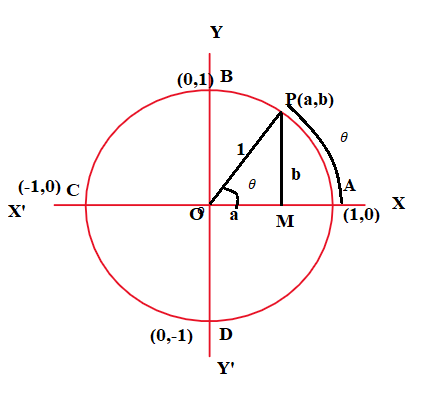December 8, 2023

# List of Trigonometric Identities

Trigonometric identities  are formulas that involve Trigonometric functions. Trigonometry is a branch of  mathematics that deals with the study of relationships between lengths and angles of triangles.  The early applications of the trigonometric functions were to surveying, navigation, and engineering. These functions  also play an important role in the study of all sorts of vibratory phenomena-sound, light, electricity, etc.

Contents

# Trigonometric Ratios

Let us take  a right triangle ABC  as shown below.

The trigonometric ratios of the angle A  in right triangle ABC  are defined   as follows :

Note that the ratios cosec A, sec A and cot A are respectively, the reciprocals of the ratios sin A, cos A and tan A. So, the trigonometric ratios of an acute angle in a right triangle express the relationship between the angle and the length of its sides. These are trigonometric ratios for with respect to acute angle A. However, these ratios can be extended to other angles also.

## Trigonometric ratios of some specific angles

 not defined not defined 2 2 not defined not defined 0

Remark : From the table above you can observe that as ∠ A increases from 0° to  90°, sin A increases from 0 to 1 and cos A decreases from 1 to 0.

### Trigonometric Ratios of Complementary Angles

#### Trigonometric Identities

An equation involving trigonometric ratios  of an angle is called a trigonometric identity, if it is   true for all values of the angle(s) involved. Some basic trigonometric identities are :

(i)

(ii)

(iii)

These are the trigonometric ratios of acute angles as the ratio of the sides of a right angled triangle.  Further the concept of trigonometric ratios  is  extended to  the trigonometric ratios to any angle in terms of radian measure and study them as trigonometric functions.

##### Trigonometric functionsConsider a unit circle with centre 0. In right angle triangle OMP ,

and  . Since

triangle OMP is a right triangle , we have

Now, if we take one complete revolution from the point P, we again come back to
same point P. Thus, we also observe that if increases (or decreases) by any integral
multiple of

, the values of sine and cosine functions do not change. Thus,

and

Further

 , if i.e. when is an integral multiple of

and

 , if , i.e. vanishes when is an odd multiple of

Thus

and

Other trigonometric functions are

, , where
is any integer

,

, where is any integer

, , where
is any integer

,

, where is any integer

Therefore we have the following identities

###### Trigonometric ratios of higher angles:
 Degree Radian sin 0 1 0 -1 0 cos 1 0 -1 0 1 tan 0 1 not defined 0 not defined 0 cot not defined 1 0 not defined 0 not defined sec 1 2 not defined -1 not defined 1 cosec not defined 2 1 not defined -1 not defined

Sign of trigonometric functions in different quadrants:

 I II III IV + + – – + – – + + – + – + + – – + – – + + – + –

Note: The signs (positive or negative) of the trigonometric ratios in the four quadrants can be remembered as
shown below.

 I II III IV All Silver Tea Cups
•  In quadrant I, all are positive.
• In quadrant II, only sine (and its reciprocal cosec) is positive.
•  In quadrant III, only tangent (and its reciprocal cot) are positive.
•  In quadrant IV, only cosine (and its reciprocal sec) is positive.

Trigonometric Functions of Sum and Difference of Two Angles

• If none of the angles and is an odd multiple of , then
• If none of the angles and  is an odd multiple of , then

• If none of the angles and is a multiple of , then

• If none of the angles and  is a multiple of , then

•   , if , n is  an integer

• where n is an integer

https://youtu.be/lsFh8MHqGTk#### Bina singh

View all posts by Bina singh →
Last Minute Preparation Tips for SSC CGLTier II 2023 ssc chsl 2023 tier 1 cut off NIRF Rankings 2023 : Top 10 Engineering colleges in India CBSE Compartment Exam 2023 Application Form SSC CGL 2023 Notification: Important Dates